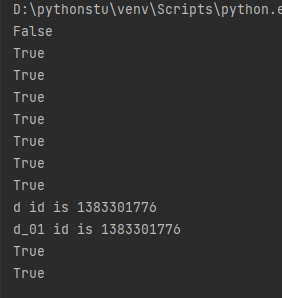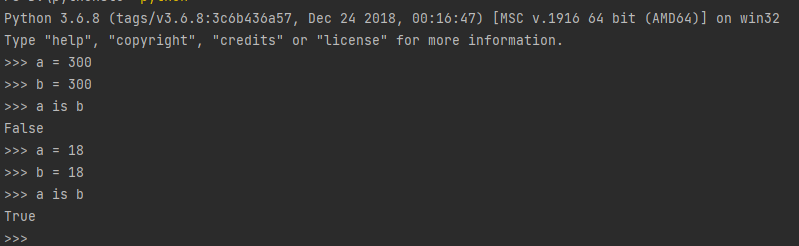## 比较与身份运算符有哪些

== 判断是否等于 a == b
! = 判断是否不等于 a != b
> 判断是否大于 a > b
< 判断是否小于 a < b
>= 判断是否大于等于 a >= b
<= 判断是否小于等于 a<= b
<> 判断是否不等于 a<> b
is 判断两个对象存储单元是否相同 a is b
is not 判断两个对象存储单元是否不同 a is not b

`<>`在Python3中已经弃用了现在仅仅能在Python2中使用

``````# coding:utf-8

a = 1
b = 2.2
c = 0
d = 18
d_01 = 18
e = -3
f = 300
f_01 = 300

print(a == b)
print(a != b)
print(a < b)
print(a > e)
print(d >= b)
print(d >= d_01)

print(d == d_01)
print(d is d_01)
print('d id is',id(d))
print('d_01 id is',id(d_01))
print(f == f_01)
print(f is f_01)
``````
• 1
• 2
• 3
• 4
• 5
• 6
• 7
• 8
• 9
• 10
• 11
• 12
• 13
• 14
• 15
• 16
• 17
• 18
• 19
• 20
• 21
• 22
• 23
• 24``````>>> a = 300
>>> b = 300
>>> a is b
False
>>> a = 18
>>> b = 18
>>> a is b
True
``````
• 1
• 2
• 3
• 4
• 5
• 6
• 7
• 8## 总结

• 在Python中0~255提前定义到了内存中
• 在Python原生编译器中如果我们定义一个大于255的变量那么后续定义相同值的变量不会指向之前我们定义的内存地址